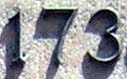## Tuesday, December 14, 2010

### 173

173 is a prime number. It is a Sophie Germain prime because 2 x 173 + 1 = 347, which is also prime.

173 has a representation as a sum of two squares: 173 = 22 + 132. It is the sum of the squares of two Fibonacci numbers, 2 and 13.

173 is the hypotenuse of a primitive Pythagorean triple: 1732 = 522 + 1652.

173 is the only prime whose sum of cubed digits equals its reversal: 13 + 73 + 33 = 371.

173 is the largest known prime whose square (29929) and cube (5177717) have no digits in common.

173 = 1 + 22 + 23 + 25 + 27, where the exponents are consecutive primes.In the 1993 movie The Sandlot, The Beast (a ferocious dog) is said to have eaten 173 guys.